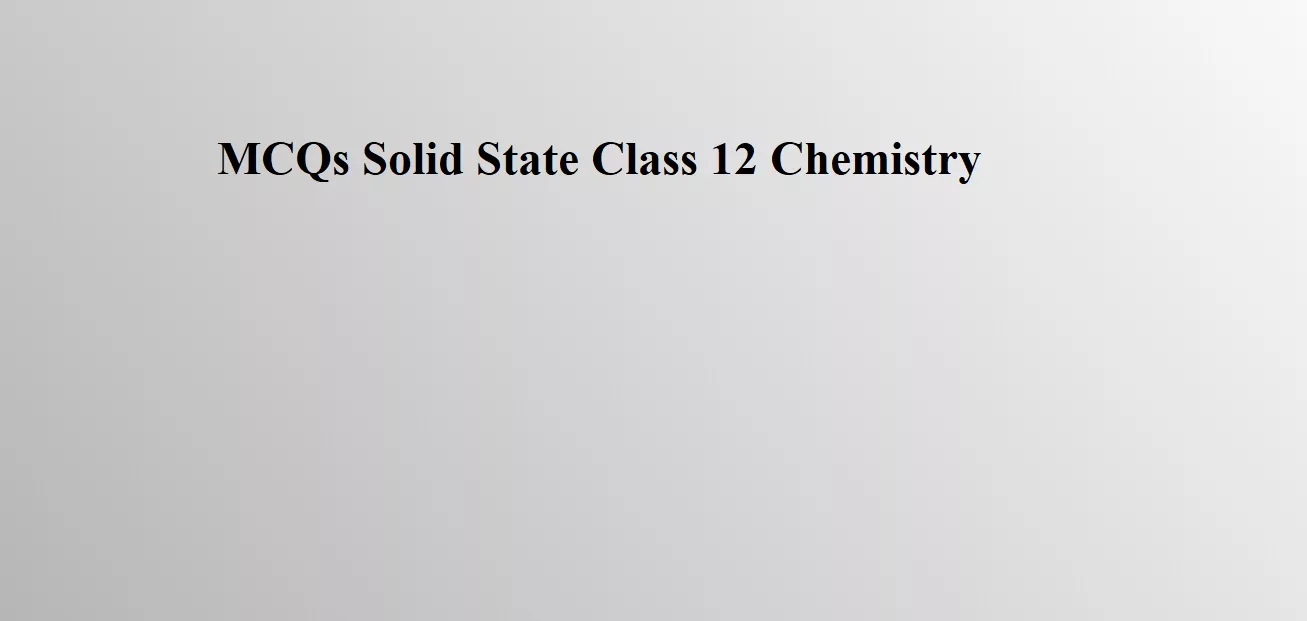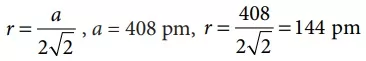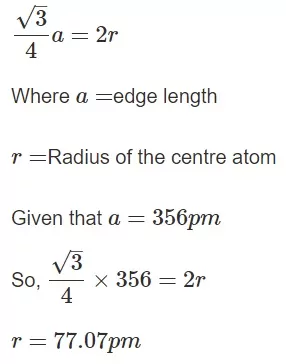# MCQs Solid State Class 12 ChemistryMCQs Solid State Class 12 Chemistry

Que 1. The total number of tetrahedral voids in the face-centered unit cell is
(a) 6                            (b) 8                            (c) 10                           (d) 12
Ans 1. (b) 8
Reason: No. of atoms per unit cell in face-centered unit cell = 4
We know for N atoms there are 2 N tetrahedral voids
∴ No. of tetrahedral voids = 2 × 4 = 8

Que 2. A compound is formed by cation C and anion A. The anions form hexagonal close-packed (hcp) lattice and the cations occupy 75% of octahedral voids. The formula of the compound is
(a) C4A3                        (b) C2A3                               (c) C3A2                        (d) C3A4
Ans 2. (d)
Reason: Number of atoms per unit cell in hcp = 6 Number of octahedral void in hcp = 6
Number of anions per unit cell = 6
Number of octahedral voids occupied by cations = 6 × 75/100 = 9/2
Formula of compound = C9/2 A6 = C3A4

Que 3. A metal crystallises with a face-centered cubic lattice. The edge of the unit cell is 408 pm. The diameter of the metal atom is
(a) 288 pm                     (b) 408 pm              (c) 144 pm                     (d) 204 pm
Ans 3. (a)
Reason: For a face centred cubic structure (fcc)Diameter = 2r = 2 × 144 = 288 pm

MCQs Solid State Class 12 Chemistry

Que 4. Lithium metal crystallizes in a body-centered cubic crystal. If the length of the side of the unit cell of lithium is 351 pm, the atomic radius of lithium will be
(a) 151.8 pm                        (b) 75.5 pm       (c) 300.5 pm              (d) 240.8 pm
Ans 4.  (a)
Reason: Since Li crystallizes in body-centered cubic crystal, atomic radius,
Given a = 351 pm

Que 5. Which one of the following metal oxides is antiferromagnetic in nature
(a) MnO2                    (b) TiO2                      (c) VO2                       (d) CrO2
Ans 5. (a)
Reason: MnO2 is antiferromagnetic

Que 6. In the calcium fluoride structure, the coordination number of the cation and anions are respectively.
(a) 6, 6                        (b) 8, 4                                    (c) 4, 4                                     (d) 4, 8
Ans 6. (b) 8, 4
Reason: The Ca2+ ions are arranged in (ccp) arrangement, i.e. Ca2+ ions are present at all corners and that the centre of each face of the cube. The fluoride ions occupy all the tetrahedral sites. This is an 8:4 arrangement i.e., each Ca2+  ion is surrounded by 8 F ions and each F ion by four Ca2+ ions.

MCQs Solid State Class 12 Chemistry

Que 7. An element (atomic mass 100g/mol) having bcc structure has unit cell edge 400 pm. Then density of the element is
(a) 10.376 g / cm3
(b) 5.188 g / cm3
(c) 7.289 g / cm3
(d) 2.144 g / cm3
Ans 7. (b) 5.188 g / cm3
Reason: The density of an element can be calculated using the formula

Que 8. In diamond, carbon atom occupies FCC lattice points as well as alternate tetrahedral voids. If the edge length of the unit cell is 356 pm, then the radius of the carbon atom is
(A) 77.07 pm
(B) 154.14 pm
(C) 251.7 pm
(D) 89 pm
Ans 8. (a)
Reason: In a diamond structure, there are atoms at each corner and alternate tetrahedral voids. Consider the body diagonal of the unit cell  (cube with side a). It corresponds to 8R, and the length  of the body diagonal isMCQs Solid State Class 12 Chemistry